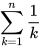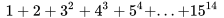# heroatrangi

Lv10

## Guru Gobind Singh Indraprastha University - GGSIPU

10 Followers
0 Following
90 Helped

Published1454

### Subjects

Answer:Assuming the pressure remain constant. V1/T1 = V2/T2 50/(273+25)= V2/(2...
Answer: e^lnx Let e^lnx = y ln(e^lnx) = lny lnX = lnY X= Y Thus e^lnX = X
Answer: Sin⁴x - Cos⁴X (Sin²X+ Cos²X)(Sin²X- Cos²X) -(Cos²X- Sin²X) -Cos2X
Answer: Sin⁴x- Cos⁴X =(Sin²X+Cos⁴X)(Sin²X- Cos²X) =-(Cos²X-Sin²X) =-Cos2X
Answer:X²-x-6 = X²-6x+x-6 = X(X-6)+1(X-6) = (X-6)(X+1)
Answer: Fractional notation for 37.5% = 37.5/100 =375/100

How do you expand the summation notation,?

How do you write the expression,in summation notation?

Answer: n=nE(n^(n-1))n=1 Where E represents summation.
Answer: = -√3sinx +cosxDividing & multiplying by 2 =2((-√3sinx)/2 +(1/2) c...
Answer: Log(12) Log(2² ×3) Log(2²) +log(3) 2log2 +log(3)
Answer: Sec(π/4) = 1/cosine(π/4) =1/(1/√2)) =√2
Answer: f(a+h) = (a+h)²-2(a+h)+3 f(a) = a²-2a+3 f(a+h)-f(a) = a²+h²+2ah-2a-2h ...
Answer: Let log(27)9 = x9 = 27^x 3² = 3^(3x) 2= 3x X= 2/3
Answer: Sin X= 1/2 X= 30°